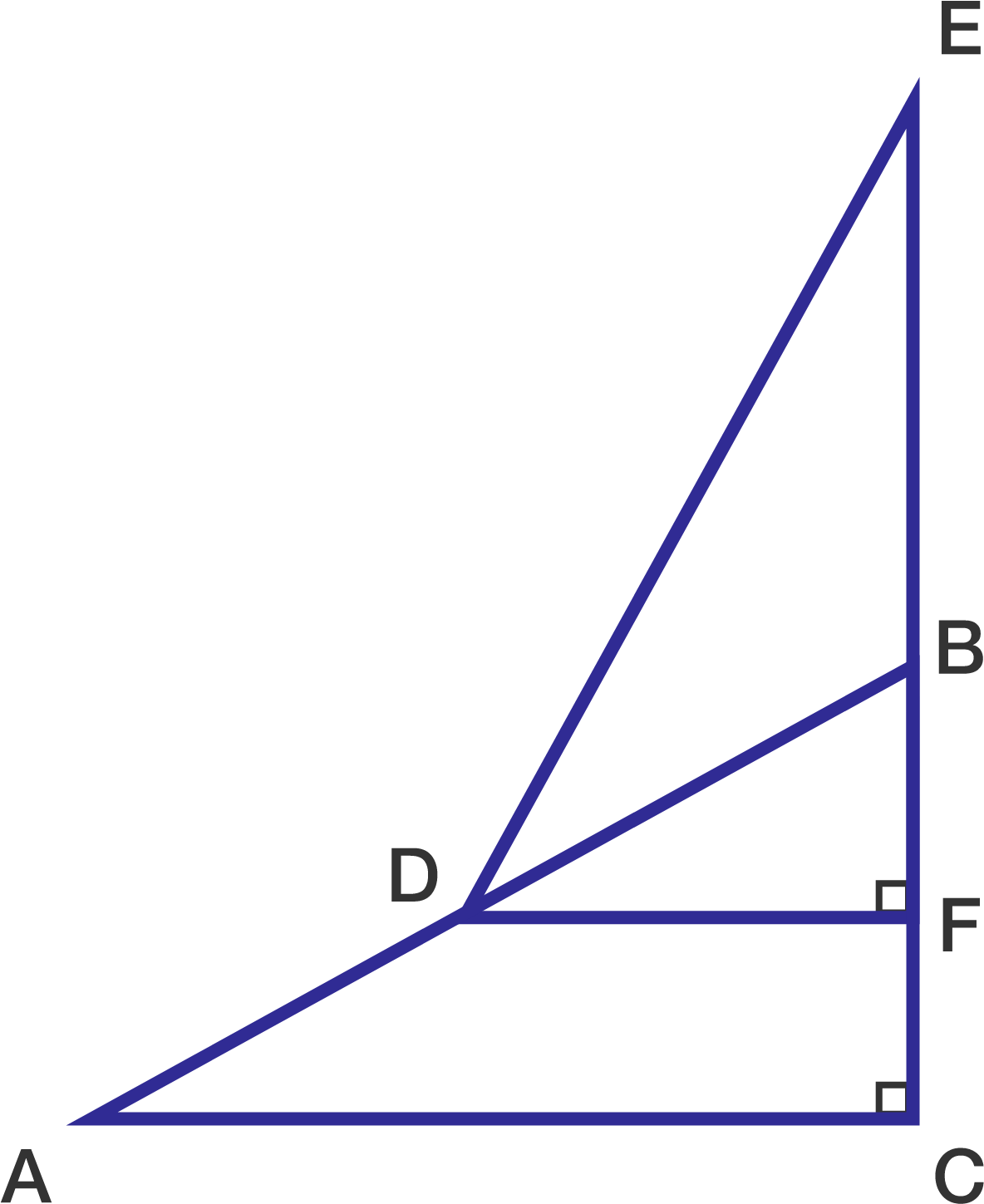# A problem by Efren MedalloIn the figure, $\Delta ABC \cong \Delta EDF$ with $\overline{AC} = \overline{EF} = 8$.

If $\Delta DEF$ is positioned in such a way that $D$ lies along $\overline{AB}$ and the area of polygon $ADEC$ is $28,$ what is the length of $\overline{FC}?$

×